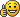## Make a sum in thyme

### Make a sum in thyme

for(n, (i)=>{ . . . })
How can I make a sum with the for-function?

for examlpe this simple sumI'm asking you because for example if I type "for(10, (i)=>{i})" it just shows the 10th member:
0 + 1 + 2 + 3 + 4 + 5 + 6 + 7 + 8 + 9 => it just shows the 10th member (the 9) as a result but not the whole sum.

So again... How can I make a sum with the for-function?

Thanks- - - - -

I just found out how it works. If anyone needs it, here's the thyme code:

Code: Select all
`scene.my.sum = (n) => {    value := 0;    for(n, (i)=>{value = value + i});    value }`

For example:
scene.my.sum(6) = 15 (because of 0 + 1 + 2 + 3 + 4 + 5)

I will extend this idea to make a general sum function so you can add everything you want.KarateBrot

Posts: 825
Joined: Mon Aug 31, 2009 7:32 pm
Location: Germany

### Re: Make a sum in thyme

To add the values in an array, use this (based on your code just now):
Code: Select all
`scene.my.sum = (length, array) =>{    value := 0    for(length, (i)=>{value = value + array(i)})    value}`

To add consecutive numbers from 0, I suggest you use this:
Code: Select all
`scene.my.sum = (n) => {(n)*(n-1)/2.0}`
Last edited by immibis on Fri Oct 09, 2009 1:18 am, edited 1 time in total.
Thymechanic
immibis

Posts: 87
Joined: Wed Sep 30, 2009 12:24 am

### Re: Make a sum in thyme

thanks for your replyi've got one question: what is the bracket of array[i] for? i only know array(i)

- - - - -

Code: Select all
`scene.my.sum = (n) => {(n)*(n-1)/2.0}`

i know this formula but i just wanted to give an example so i don't want to only calculate 1+2+3+4+...+nKarateBrot

Posts: 825
Joined: Mon Aug 31, 2009 7:32 pm
Location: Germany

### Re: Make a sum in thyme

Sorry, that should be array(i). I've edited my post.
Thymechanic
immibis

Posts: 87
Joined: Wed Sep 30, 2009 12:24 am